## Characterizing anomalous diffusion and ergodicity breaking from single trajectories

In this notebook you will find the basic tools to learn how to deal in practice with the topic of diffusion. First, few simple comments about the andi_datasets package. We will use this python package to create trajectories of different anomalous diffusion models. You can find all details in the documentation webpage.

Let’s start by importing the models_theory module and checking the available anomalous diffusion models.

from andi_datasets.models_theory import models_theory
dimensions = 1
models = models_theory()._oneD()

available_models = inspect.getmembers(models, inspect.ismethod)

print('The availailabe models for generating trajectories in '+str(dimensions)+'D  are:\n')
for x in available_models:
print('- ' + x + '\n')
The availailabe models for generating trajectories in 1D  are:

- attm

- ctrw

- fbm

- lw

- sbm


The andi-datasets package allows us then to generate trajectories according to these five models. We will learn more about them along the notebook. To generate a single trajectory of, for instance, Fractional Brownian motion (fbm) with anomalous diffusion exponent $$\alpha=0.5$$ we just need to do:

T = 200 # Length of the trajectories
alpha = 0.5 # Anomalous diffusion exponent
model = 2 # This corresponds to the index of fbm in the previous list

traj = models.fbm(T = T, alpha = alpha)
plt.plot(traj)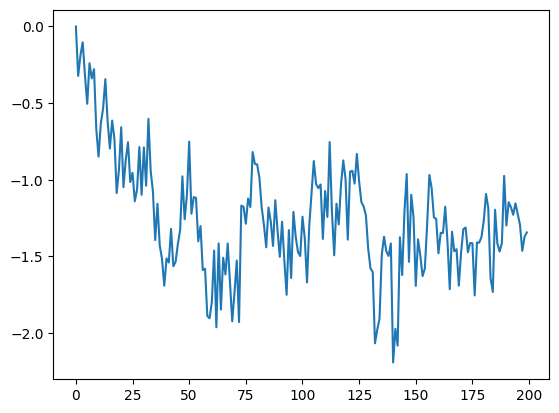Moreover, the andi_datasets library allows us to create bigger datasets of trajectories with the datasets_theory module. Below we show how to do this. There, dataset is a numpy matrix whose first two columns indicate the exponent and model of each trajectory (now labels) and the rest of the matrix is the trajectory itself (now trajs). Let’s check how the trajectories look like:

from andi_datasets.datasets_theory import datasets_theory
DT = datasets_theory()

N = 5 # Number of trajectories

dataset = DT.create_dataset(T = T, N_models = N, exponents = alpha, models = )

labels = dataset[:,:2]
trajs = dataset[:,2:]

# dispay a few
for traj in trajs:
plt.plot(traj)
plt.xlabel('time')
plt.ylabel('position')
Text(0, 0.5, 'position')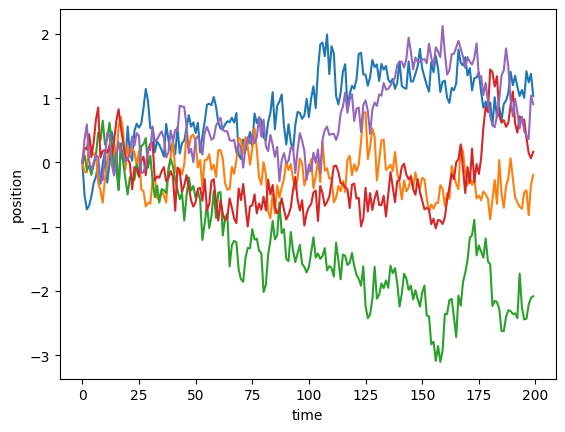## Anomalous diffusion exponent

In this section we will show the basic tools to asses the anomalous diffusion exponent of a set of trajectories.

### Time averaged mean squared displacement (TA-MSD)

We will now create a function that calculates the TA-MSD for a given trajectory:

(Note that this function calculates the TA-MSD for a single trajectory. Using the theoretical definition and the function below, can you rewrite the funcion such that it calculates the TA-MSD for a set of trajectories in an efficient way?)

def TAMSD(traj, t_lags):
'''
Calculates the time average mean squared displacement of a set of trajectories
Inputs: - traj: trajectory to calculate TA-MSD
- t_lags: time lags used for the TA-MSD
Outputs: - TA-MSD
'''
tamsd = np.zeros_like(t_lags, dtype= float)
for idx, t in enumerate(t_lags):
for p in range(len(traj)-t):
tamsd[idx] += (traj[p]-traj[p+t])**2
tamsd[idx] /= len(traj)-t
return tamsd

Let’s use the dataset we created above and calculate the TAMSD of each trajectory and plot it in log-scale. As the trajectories have 200 points, let’s choose the time lages $$\Delta \in [1,20]$$. In addition, we will also plot two lines: one linearly proportional to the $$\Delta$$ and one proportional to $$\Delta^\alpha$$. This will give you information about the ergodicity! Can you tell from here if fbm is ergodic or not?

t_lags = np.arange(1, 101)

for traj in trajs[:5]:
tamsd = TAMSD(traj, t_lags)
plt.loglog(t_lags,tamsd)

plt.loglog(t_lags, 0.1*t_lags, c = 'k', label = r'$\sim \Delta$')
plt.loglog(t_lags, 0.1*t_lags**alpha, c = 'k', ls = '--', label = r'$\sim \Delta^\alpha$')
plt.xlabel(r'Time lag ($\Delta$)')
plt.ylabel('TA-MSD')
plt.legend()
<matplotlib.legend.Legend>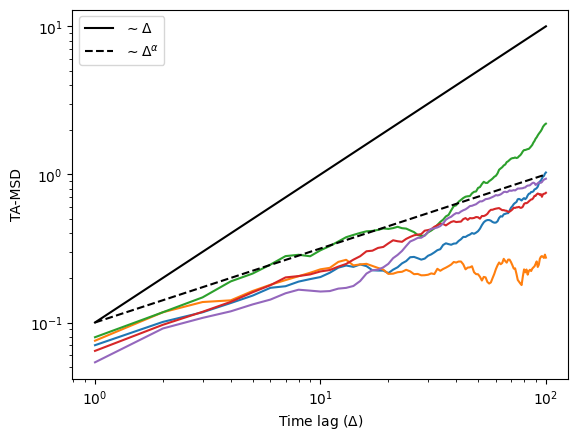We can also calculate the ensemble averaged (EA-MSD) and time-ensemble averaged mean squared displacement (TEA-MSD):

## EA-MSD ##
eamsd = np.mean(trajs**2, axis = 0)

plt.loglog(eamsd)
plt.loglog(np.arange(1,T), 0.1*np.arange(1,T), c = 'k', label = r'$\sim$ time')
plt.loglog(np.arange(1,T), 0.1*np.arange(1,T)**alpha, c = 'k', ls = '--', label = r'$\sim$ time$^\alpha$')
plt.xlabel(r'time')
plt.ylabel('EA-MSD')
plt.legend()
<matplotlib.legend.Legend>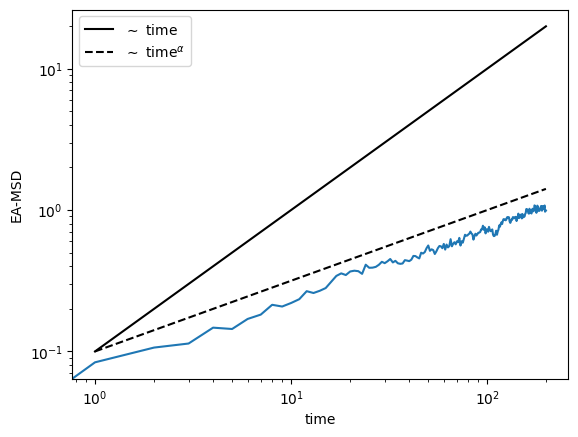## TEA-MSD ##
t_lags = np.arange(1, 21)
teamsd = np.zeros_like(t_lags, dtype = 'float64')
for traj in trajs:
teamsd += TAMSD(traj, t_lags)
teamsd /= N

plt.loglog(t_lags,teamsd, lw = 3)
plt.loglog(t_lags, 0.07*t_lags, c = 'k', label = r'$\sim \Delta$')
plt.loglog(t_lags, 0.07*t_lags**alpha, c = 'k', ls = '--', label = r'$\sim \Delta^\alpha$')
plt.xlabel(r'time lag ($\Delta$)')
plt.ylabel('TEA-MSD')
plt.legend()
<matplotlib.legend.Legend>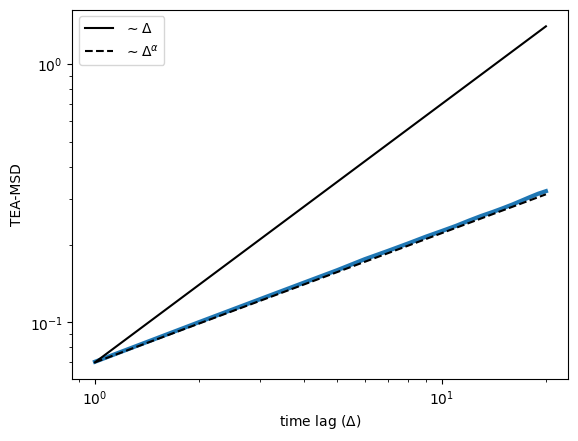A visual inspection seems to tell us that FBM is ergodic! To confirm it, let’s fit the TA-MSD and check if we recover the anomalous diffusion exponent we set above. For that, we will use a fitting function from numpy, forcing a linear fit:

fitted_alpha = []
for traj in trajs:
tamsd = TAMSD(traj, t_lags)
fitted_alpha.append(np.polyfit(np.log(t_lags), np.log(tamsd),1))

plt.scatter(range(N), fitted_alpha, label = r'Fitted $\alpha$')
plt.axhline(alpha, label = r'True $\alpha$')
plt.legend()
plt.xlabel('Trajectory number')
plt.ylabel(r'$\alpha$')
plt.ylim(0,1)
(0.0, 1.0)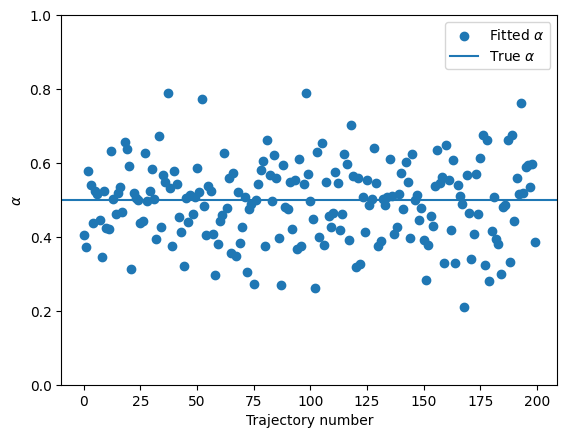The TA-MSD fit seemed to work, but rather poorly… What can be improved? Solve the exercises to learn about it!

### Trajectories with localization error

The trajectories we used aboved were examples from a purely theoretical fbm. However, in real life things are not so clean and in many experiments we have the appearance of localization noise. A simple way of simulating this is to add Gaussian noise to each of the positions of a trajectory. Here is an example with noise with standard deviation $_{} = 0.5$:

sigma_n = 0.3
noisy_trajs = trajs + np.random.randn(N,T)*sigma_n

plt.plot(trajs[0,:], label = 'Original trajectory')
plt.plot(noisy_trajs[0,:], label = 'Noisy trajectory', alpha = 0.8)
plt.xlabel('Time')
plt.ylabel('Position')
plt.legend()
<matplotlib.legend.Legend>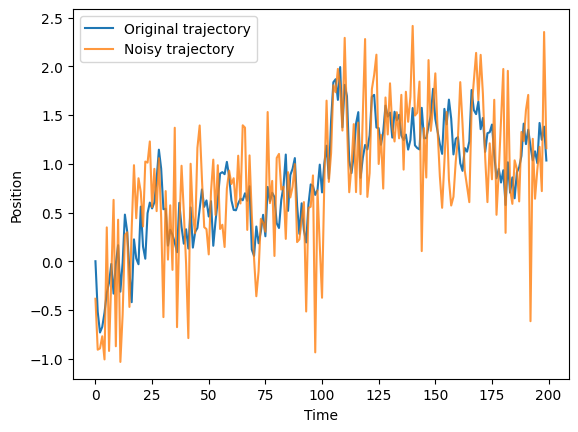Let’s see what happens when we try to predict the anomalous diffusion exponent for this noisy trajectories:

fitted_alpha_noisy = []
for traj in noisy_trajs:
tamsd = TAMSD(traj, t_lags)
fitted_alpha_noisy.append(np.polyfit(np.log(t_lags), np.log(tamsd),1))

plt.scatter(range(N), fitted_alpha, label = r'Fitted $\alpha$ (normal trajs.)')
plt.scatter(range(N), fitted_alpha_noisy, label = r'Fitted $\alpha$ (noisy trajs.)')

plt.axhline(alpha, label = r'True $\alpha$')
plt.legend()
plt.xlabel('Trajectory number')
plt.ylabel(r'$\alpha$')
plt.ylim(0,1)
(0.0, 1.0)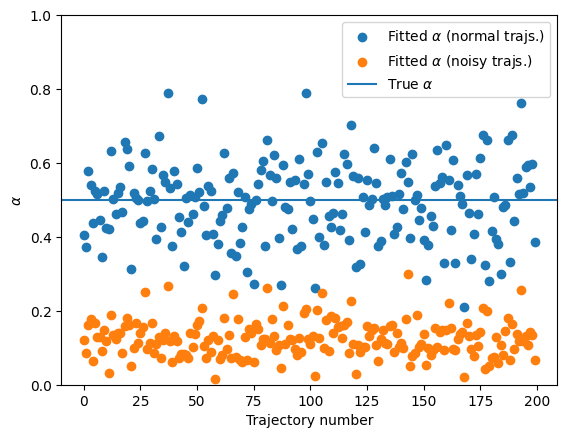You can see that the noise has largely erased the information of the anomalous diffusion exponent!! This shows the need for better techniques if one wants to correctly characterize a trajectory. In the last part of this notebook we will show a novel approach based on ML, with much better results! Having an independent estimation of the noise allows for a correction and provides a better quantification of $$\alpha$$:

fitted_alpha_noisy_corr = []
for traj in noisy_trajs:
tamsd = TAMSD(traj, t_lags) - 2*(sigma_n**2)
i_ok = np.where(tamsd>0)
fitted_alpha_noisy_corr.append(np.polyfit(np.log(t_lags[i_ok]), np.log(tamsd[i_ok]),1))

plt.scatter(range(N), fitted_alpha, label = r'Fitted $\alpha$ (normal trajs.)')
plt.scatter(range(N), fitted_alpha_noisy, label = r'Fitted $\alpha$ (noisy trajs.)')
plt.scatter(range(N), fitted_alpha_noisy_corr, label = r'Fitted $\alpha$ (noisy trajs. with corr.)')

plt.axhline(alpha, label = r'True $\alpha$')
plt.legend()
plt.xlabel('Trajectory number')
plt.ylabel(r'$\alpha$')
plt.ylim(0,1)
(0.0, 1.0)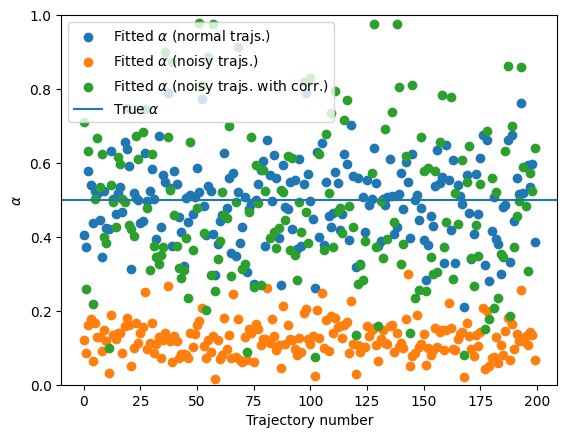## Ergodicity breaking

In the cells above we have focused on an ergodic model, FBM. However, we now know that there exist diffusion models that break ergodicity. This means that the time average mean squared displacement (TA-MSD) and the ensemble average mean squared displacement (EA-MSD) are not longer equal. Let’s explore this intriguing feature with a very well known non-ergodic model: continuous time random walk (CTRW). We will start by creating a dataset of CTRW trajectories. As we will be perfoming ensemble averages, we will need much more trajectories.

T = 200 # Length of the trajectories
N = 1000 # Number of trajectories
alpha = 0.5 # Anomalous diffusion exponent

# Now we want CTRW trajectories (see list of available models above):
model = 4

dataset = DT.create_dataset(T = T, N_models=N, exponents = alpha, models = model)
labels = dataset[:,:2]
trajs = dataset[:,2:]

This is how CTRW trajectories look like. You will recognize the characteristic waiting times.

for traj in trajs[:5,:]:
plt.plot(traj)
plt.xlabel('Time')
plt.ylabel('Position')
Text(0, 0.5, 'Position')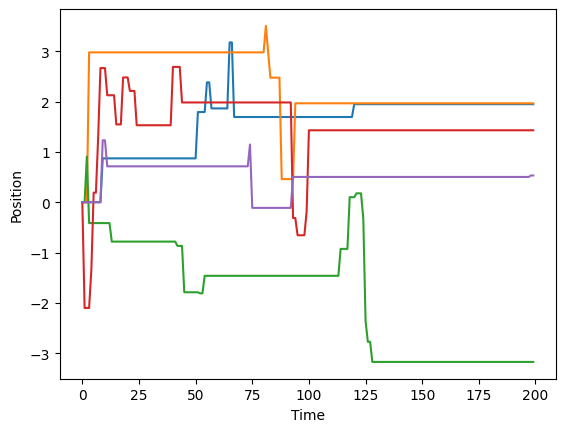### Ensemble averaged mean squared displacement (EA-MSD)

To showcase the appearance of ergodicity breaking, let’s calculate the EA-MSD and the ensemble average of the TA-MSD (TEA-MSD). For the former, if all your trajectories start at zero, it is as easy as to do the mean of the position squared! For the latter, we will use the function we define previously. We will use the same time lags we used for the FBM case.

## EA-MSD ##
eamsd = np.mean(trajs**2, axis = 0)

## TEA-MSD ##
t_lags = np.arange(1, 21)
teamsd = np.zeros_like(t_lags, dtype = 'float64')
for traj in trajs:
teamsd += TAMSD(traj, t_lags)
teamsd /= N

fig, ax = plt.subplots(1,2, figsize = (10, 5), constrained_layout = True)

ax.loglog(t_lags, teamsd)
ax.loglog(t_lags, 1.1*teamsd*t_lags, c = 'k', label = r'$\sim \Delta$')
ax.loglog(t_lags, 1.1*teamsd*t_lags**alpha, c = 'k', ls = '--', label = r'$\sim \Delta^\alpha$')
plt.setp(ax, xlabel =r'Time lag ($\Delta$)', ylabel = 'TEA-MSD')
ax.legend()

ax.loglog(eamsd)
ax.loglog(np.arange(1,T), eamsd*np.arange(1,T), c = 'k', label = r'$\sim t$')
ax.loglog(np.arange(1,T), eamsd*np.arange(1,T)**alpha, c = 'k', ls = '--', label = r'$\sim t^\alpha$')
plt.setp(ax, xlabel =r'Time', ylabel = 'EA-MSD')
ax.legend()
<matplotlib.legend.Legend>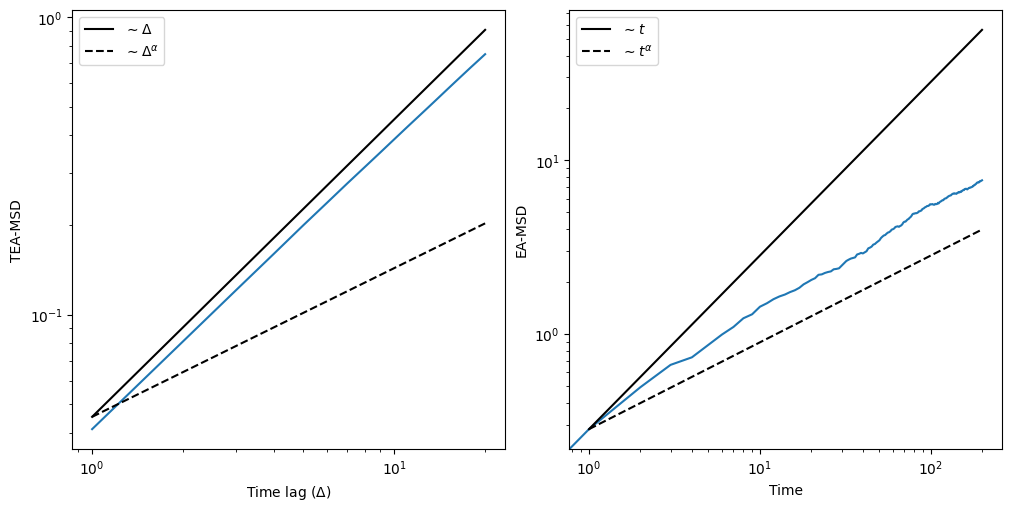As we can clearly see, the TEA-MSD is linearly proportional to the time lags $$\Delta$$ while the EA-MSD is propotional to $$t^\alpha$$, showcasing the break of ergodicity.

### Ergodicity breaking parameter

A quantitative approach to ergodicity breaking is the calculation of the ergodicity breaking parameter (EB). Here is how to calculate it:

def EB(trajs, t_lag):
eb = []
# We loop over T, which is the point at which we cut the trajectory
for T in range(t_lag+1, trajs.shape):
cut_trajs = trajs[:,:T]
# Now we calculate the TAMSD for every trajectory at the
# given t_lag
tamsd = np.zeros(trajs.shape)
for idx, traj in enumerate(cut_trajs):
for p in range(len(traj)-t_lag):
tamsd[idx] += (traj[p]-traj[p+t_lag])**2
tamsd /= len(traj)-t_lag
# Compute EB and append
eb.append(np.mean(tamsd**2)/np.mean(tamsd)**2-1)
return eb

Let’s compute the EB for similar trajectories to the ones we used in the previous section. The expected value at $$t\rightarrow\infty$$ is given by Eq.(8) of this paper and for $$\alpha = 0.5$$ we should have EB$$\approx 0.570$$. As a bonus, let’s create some FBM trajectories to check that for ergodic processes, the EB goes to zero, even when the anomalous diffusion exponent is different than one. We will use as time lag $$\Delta = 2$$. You can check the effect of varying $$\Delta$$ on the EB in the previous paper (see e.g. Fig. 2(b)).

Note: the EB is much slower because you need a couple of extra loops. If you managed to create the efficient function for the TA-MSD, now is the time to use it!

T = 200 # Length of the trajectories
N = 500 # Number of trajectories
alpha = 0.5 # Anomalous diffusion exponent

fbm = DT.create_dataset(T = T, N_models=N, exponents = alpha, models = 2)[:,2:]
ctrw = DT.create_dataset(T = T, N_models=N, exponents = alpha, models = 4)[:,2:]
EB_ctrw = EB(ctrw, t_lag = 2)
EB_fbm = EB(fbm, t_lag = 2)
plt.plot(EB_ctrw, label = 'CTRW')
plt.plot(EB_fbm, label = 'FBM')
plt.axhline(0.570, c = 'k', alpha = 0.4, label = r'EB($t\rightarrow\infty$) CTRW')
plt.ylabel('EB(t)')
plt.xlabel('Time')
plt.legend()
<matplotlib.legend.Legend>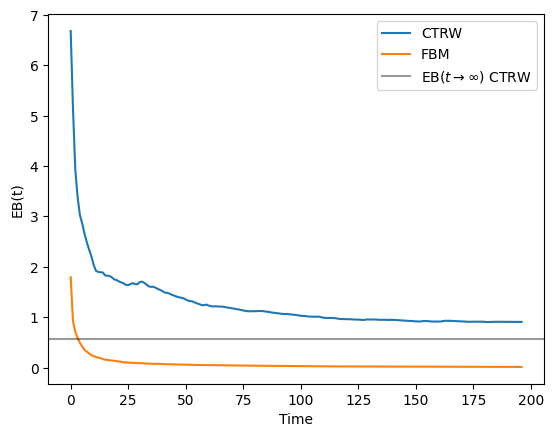As you can see, the EB for the FBM trajectories quickly goes to 0. However, for the CTRW we see a value different that zero, that will converge to the theoretical value if we consider much longer trajectories. Feel free to explore how long you have to wait until reaching such value!

## Machine learning approach to single trajectory characterization

As we just saw, analyzing diffusion processes at the single trajectory level has many problems related to, e.g. noise, trajectory length, ergodicity breaking,… In the last years we have seen how machine learning can indeed solve many of this. Here we will show an example on how to use a convolutional neural network to decide wether the anomalous diffusion exponent of a dataset of trajectories.

### Training and test dataset

Let’s start by creating the datasets that we will use for training and evaluating the trained machine. We will consider for this example a dataset of FBM trajectories, with exponents $$\in [0.2,1.4]$$ and lenght 200. For training we need a big dataset, so we will generate 10000 trajectories:

num_alphas = 100
exponents = np.linspace(0.2,1.81, num_alphas)
N_models = 100 # this is the number of trajectories per exponent, which makes a total of 10000
T = 200 # Length of the trajectories

dataset = DT.create_dataset(T = T, N_models=N_models, exponents = exponents, models = 2)

We will now transform the previous dataset to a machine learning dataset which we can then input to the neural network. The shape constraints are set by the machines we will later use. Moreover, we will split the dataset into a training and test datasets with a 80%/20% ratio. Moreover, we will use the function normalize from the andi-datasets package to ensure that all the trajectories are in the same scale. This function divides the displacements of a trajectory by their standard deviation. This changes the diffusion coefficient of any trajectory to $$D=1$$, but the anomalous diffusion exponent remains untouched. Moreover, it is also important to shuffle our dataset.

from andi_datasets.utils_trajectories import normalize

ratio = int(0.8*dataset.shape)
np.random.shuffle(dataset)
# NN inputs: trajectories
X_train = normalize(dataset[:ratio, 2:]).reshape(ratio, T, 1)
X_test = normalize(dataset[ratio:, 2:]).reshape(N-ratio, T, 1)

# NN outputs: their anomalous exponent
Y_train = dataset[:ratio, 1]
Y_test = dataset[ratio:, 1]

### Neural network architecture

from keras.models import Sequential, Model
from keras.layers import Dense, Dropout, Conv1D, Flatten, BatchNormalization
from keras.regularizers import l2 as regularizer_l2

model = Sequential()

input_shape=(T, 1),
kernel_initializer= 'uniform',
activation= 'relu', kernel_regularizer = regularizer_l2(l = 0.001)))

kernel_initializer= 'uniform',
activation= 'relu', kernel_regularizer = regularizer_l2(l = 0.001)))

kernel_initializer= 'uniform',
activation= 'relu', kernel_regularizer = regularizer_l2(l = 0.001)))

model.add(Dense(64*2, activation='sigmoid', kernel_regularizer = regularizer_l2(l = 0.001)))

model.add(BatchNormalization(axis=-1, momentum=0.99, epsilon=0.001))

As we want to extract the anomalous diffusion exponent, the output layer needs to have size one. It is also important that its activation function is linear.

model.add(Dense(1, activation='linear'))

Now that we have all the layers, we can take a look at a visual summary of it. The plot below also gives us the input/output shape for every layer!

from keras.utils.vis_utils import plot_model

plot_model(model, show_shapes=True, show_layer_names = False)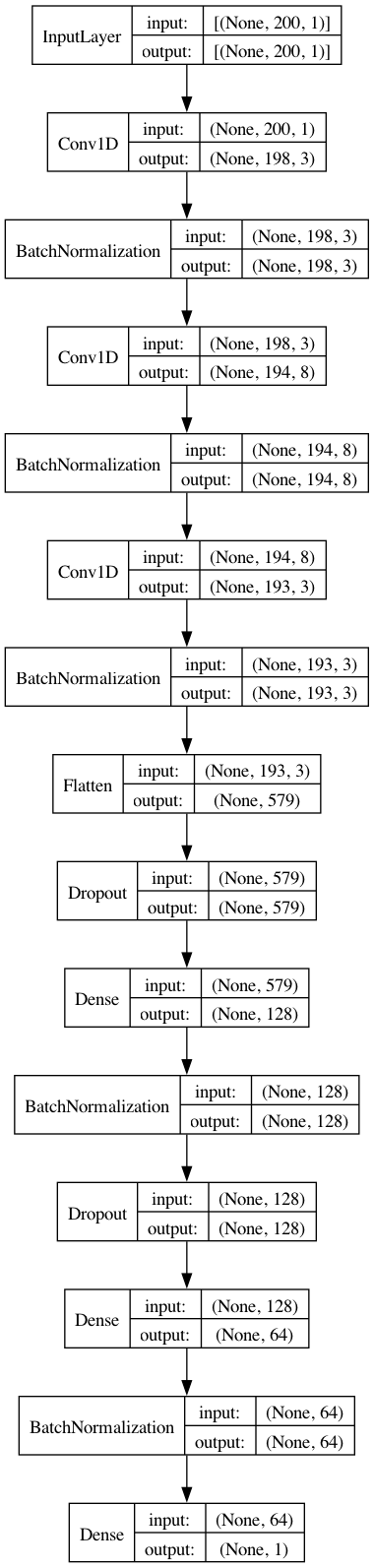Now we compile the model. For the loss function, we will use the mean absolute error (MAE). Another possible choice is the mean squared error (MSE). We will keep track of it as metric. We will use Adam as optimizer.

model.compile(loss='mean_absolute_error',
optimizer=Adam())

At last, we train!

batch_size = 200
epochs = 150

training = model.fit(X_train, Y_train,
batch_size=batch_size,
epochs=epochs,
verbose=2,
validation_data=(X_test, Y_test))

In order to see if the training worked, let’s take a look at the training and test dataset loss:

acc = training.history['loss']
val_acc = training.history['val_loss']

plt.plot(np.arange(len(training.history['loss'])), acc, label='Training loss')
plt.plot(np.arange(len(training.history['loss'])), val_acc,label='Validation loss')
plt.xlabel('Epochs')
plt.ylabel('Accuracy')
plt.legend()
<matplotlib.legend.Legend>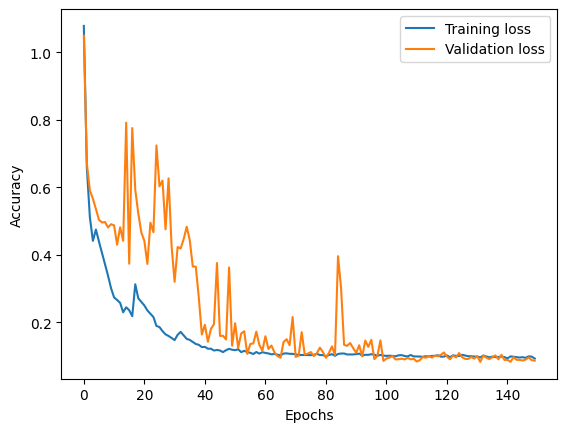Let’s create a validation dataset with few exponents and check the predictions:

validation_exponents =  np.linspace(0.5, 1.5, 4)

val_dataset = DT.create_dataset(T = T, N_models=1000, exponents = validation_exponents, models = 2)
X_val = normalize(val_dataset[:, 2:]).reshape(val_dataset.shape, T, 1)
Y_val = val_dataset[:, 1]
preds_val = model.predict(X_val)
125/125 [==============================] - 0s 2ms/step

Now we can plot de distribution of predicted exponents for each of the groundtruth ones:

fig, ax = plt.subplots(figsize = (15,3))
for idx, u in enumerate(np.unique(Y_val)):
ax.hist(preds_val[Y_val == u], facecolor = f'C{idx}', bins = 100, alpha = 0.4, label = 'Predicted value' if idx == 0 else '')
plt.axvline(u, ls = '--', c = f'C{idx}', label = 'True value' if idx == 0 else '')
plt.legend()
plt.xlabel('Anomalous exponent')
Text(0.5, 0, 'Anomalous exponent')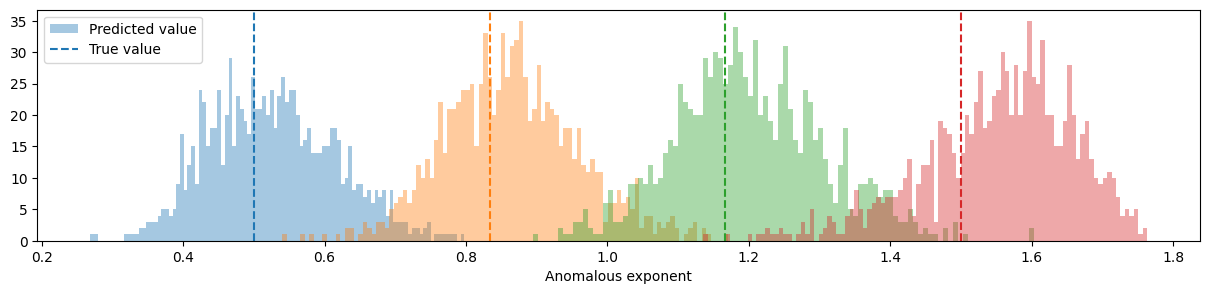As we can see the methods is able to correctly predict the exponent! The bigger the error, the more spread this distributions will be. Can you improve the model? Go for it!

### Extracting other parameters with ML

Now that we defined our network, it is really easy to transform it for any other task. For instance, let’s see how to create a machine that distinguishes between ergodic and non-ergodic trajectories. First we need to create a new dataset with all the andi-datasets diffusion models. Due to the constraints of the package, we will need to create the subdiffusive and superdiffusive datasets separately and then merge them:

N = 200 # this is the number of trajectories per exponent, which makes a total of 10000
T = 200 # Length of the trajectories

subdiffusive_exponents = np.arange(0.2, 1, 0.2) # We only consider subdiffusion
subdiffusive_models = [0, 1, 2, 4] # All models but LW

sub_dataset = DT.create_dataset(T = T, N_models = N, exponents = subdiffusive_exponents, models = subdiffusive_models)

superdiffusive_exponents = np.arange(1, 2, 0.2) # We only consider superdiffusion
superdiffusive_models = [2, 3, 4] # All models but CTRW and ATTM

super_dataset = DT.create_dataset(T = T, N_models = N, exponents = superdiffusive_exponents, models = superdiffusive_models)

dataset = np.vstack((sub_dataset, super_dataset))

The labels now need to be transform such that for ATTM, CTRW and SBM we have 0 (non-ergodic) and for FBM and LW we will have 1 (ergodic):

ratio = int(0.8*dataset.shape)
np.random.shuffle(dataset)
# NN inputs: trajectories
X_train = normalize(dataset[:ratio, 2:]).reshape(ratio, T, 1)
X_test = normalize(dataset[ratio:, 2:]).reshape(N-ratio, T, 1)

# NN outputs
# First we take the models
Y_train = dataset[:ratio, 0]
Y_test = dataset[ratio:, 0]

where_ergodic = np.argwhere((Y_train == 2) | (Y_train == 3))
where_nonergodic = np.argwhere((Y_train == 0) | (Y_train == 1) | (Y_train == 4))
Y_train[where_ergodic] = 0
Y_train[where_nonergodic] = 0

where_ergodic = np.argwhere((Y_test == 2) | (Y_test == 3))
where_nonergodic = np.argwhere((Y_test == 0) | (Y_test == 1) | (Y_test == 4))
Y_test[where_ergodic] = 0
Y_test[where_nonergodic] = 0

Finally, we can use the same architecture as before, but will need to change the last layer to two neurons with softmax activation, as e.g.:

model.add(Dense(2, activation='softmax'))

Now you are on your own, can you train this network?